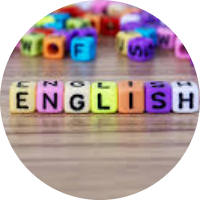# Fun with Mathematics

This set of MCQs helps you brush up on important math topics and prepare you to dive into skill practice.

Start Quiz

Three points A, B, and C have coordinates (0, 4), (6, 2), and (10, 4), respectively. Then ∠ABC equals _____.

135

145

125

165

Suppose a satisfies the equation 4 = 𝑎 + 𝑎 ^−1. What is the value of 𝑎^ 4 + 𝑎^ −4?

182

183

192

194

Three points A, B, and C have coordinates (0, 4), (6, 2), and (10, 4), respectively. Then ∠ABC equals _____.

135

145

125

165

Which one of these numbers must be placed in the middle (3rd) if they are to be arranged in increasing or decreasing order?

(√13)/2

(√11-√13)/2

(√11+√13)/2

11

If the polynomial p(y)=−3y^3+5y^2+ky+m, when divided by (y−1) and (y+1), leaves the remainder 13 and 6 then the remainder when it is divided by (y+3) is,

121

111

11

101

The numbers 𝑎1, 𝑎2, 𝑎3, and 𝑎4 are drawn one at a time from the set {0, 1, 2, …, 9}. If these four numbers are drawn with replacement, what is the probability that 𝑎1𝑎4 − 𝑎2𝑎3 is an even number?

0.5

0.75

0.25

0.33

If the angles of a triangle are in the ratio of 4:5:2, the triangle is :

An acute-angled triangle

An obtuse-angled triangle

A right-angled triangle

An isosceles triangle

A function f is defined by f(x)=6x−53. What is the value of f(11)?

10

-13

20

13

In a right-angled △ABC,∠ACB=60^∘,∠BAC=30^∘ , and ∠ABC=90^∘. If AC=8 cm, then the length of BC is cm.

8

2

4

16

Which of the following equations represents a straight line passing through the points (0,0), (−8,8), and (8,−8).

x-y=0

x + y=0

x+2y=0

x-2y=0

Quiz/Test Summary
Title: Fun with Mathematics
Questions: 10
Contributed by: